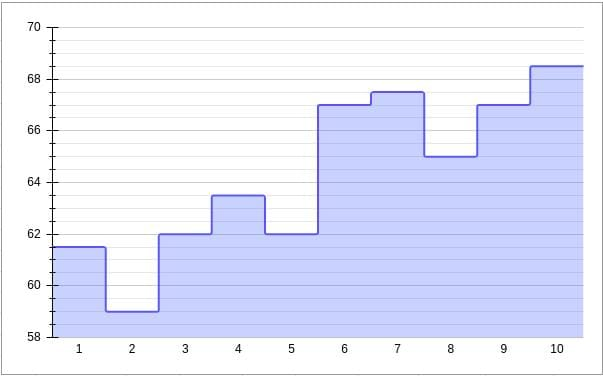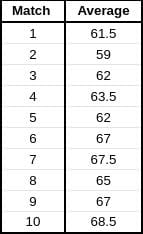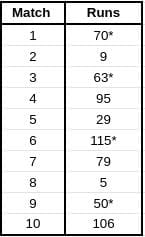Given below is a chart showing the average of a batsman, which gets updated after every match that he plays in a tournament. Before the start of the tournament, wherein he played 10 matches, he averaged 58 in 20 innings. He got out in each one of them. The batsman played the tournament with an intent to remain not out in as many innings he can. In this tournament, the highest he could score was 115.Consider, average of a batsman= Total runs scored in his career/Total time he was out
NOTE: Averages shown in the graph above are in multiples of 0.5
Q. What was the highest score of the batsman in the innings where he got out?

### Related TestBakliwal Institute Jan 18, 2022It is clear that a batsman can score a minimum of 0 runs in an innings. When he remains not out, his average remains the same (with 0 runs) and does not decrease. Therefore, if a batsman is not out in an innings, his average after the innings will not drop in any case.
Using this, we can infer that the batsman must have been out in 2nd, 5th and 8th match. In the rest of the matches, he can either be out or not out, both.
It is given that before the start of the tournament, the batsman averaged 58 in 20 innings. So, his total runs= 58 x 20 = 1160After match 1
CASE 1- He is out. Total times, batsman was out = 20+1= 21.
Total runs after this match= Average after this match x Total number of times he was out= 61.5 × 21= 1291.5, which is not possible (He cannot score runs in decimal).
CASE 2- He was not out in that innings. Total times, the batsman was out = 20
Total runs after this match= Average after this match ×Total number of times he was out=61.5 x 20 = 1230, which is possible. Therefore, Case 2 is true and he scored (1230-1160)= 70 not out in the first match.
Match 2- As discussed earlier, the batsman cannot remain not out when the average drops. He must have been out in this match.
Total times, the batsman was out = 20+1= 21.
Total runs after this match= Average after this match ×Total number of times he was out= 59×21= 1239.
So, he scored a total of 1239- 1230= 9 runs in this match.
Match 3-
CASE 1- The batsman was out. Total number of times he was out= 21+1= 22.
Total runs after this match= Average after this match ×Total number of times he was out= 62 x 22 = 1364.
Runs scored if he was out= 1364- 1239= 125, which is not possible because his highest score in the tournament was that of 115.
CASE 2- The batsman remained not out. Total number of times he was out= 21.
Total runs after this match= Average after this match ×Total number of times he was out=62 x 21 = 1302.
Runs scored in this match= 1302- 1239= 63 not out.
Match 4-
CASE 1- The batsman was out. Total number of times he was out= 21+1= 22.
Total runs after this match= Average after this match ×Total number of times he was out= 63.5 ×22= 1397.
Runs scored in this match= 1397-1302=95.
CASE 2- The batsman remained not out. Total number of times he was out= 21.
Total runs after this match= Average after this match ×Total number of times he was out= 63.5 ×21= 1333.5, which is not possible.
Hence, case 1 is true and he scored 95 in this match before getting out.
Match 5- It is clear that the batsman was out in this match as there is a drop of average after this match. So, total number of times the batsman was out after this match= 22+1= 23.
Total runs after this match= Average after this match ×Total number of times he was out= 62 ×23= 1426.
Runs scored in this match= 1426-1397=29.
Match 6-
CASE 1- The batsman was out. Total number of times he was out= 23+1= 24.
Total runs after this match= Average after this match ×Total number of times he was out= 67 ×24= 1608.
Runs scored in this match= 1608-1426= 182, which is not possible because his highest score was 115.
CASE 2- The batsman was not out. Total number of times he was out= 23
Total runs after this match= Average after this match ×Total number of times he was out= 67 ×23= 1541.
Runs scored in this match= 1541-1426= 115, which is possible. So, case 2 is true and he scored 115 not out in this match.
Match 7-
CASE 1- The batsman was out. Total number of times he was out= 23+1= 24.
Total runs after this match= Average after this match ×Total number of times he was out= 67.5 ×24= 1620.
Runs scored in this match= 1620-1541= 79, which is possible.
CASE 2- The batsman was not out. Total number of times he was out= 23
Total runs after this match= Average after this match ×Total number of times he was out= 67.5 ×23= 1552.5, which is not possible as runs cannot be in decimals.
So, in match 7, the batsman scored 79 before getting out.
Match 8- As discussed earlier, he was out in this match as there is a drop in average. Total number of times he was out= 24+1= 25.
Total runs after this match= Average after this match ×Total number of times he was out= 65 ×25= 1625.
Runs scored in this match= 1625-1620= 5.
Match 9-
CASE 1-  The batsman was out. Total number of times he was out= 25+1= 26.
Total runs after this match= Average after this match ×Total number of times he was out= 67 ×26= 1742.
Runs scored in this match= 1742-1625= 117, which is not possible as his highest score is of 115.
CASE 2- The batsman was not out. Total number of times he was out= 25
Total runs after this match= Average after this match ×Total number of times he was out= 67 ×25= 1675.
Runs scored in this match= 1675-1625= 50.
So, he scored 50 not out in the 9th match of the tournament.
Match 10-
CASE 1- The batsman was out. Total number of times he was out= 25+1= 26.
Total runs after this match= Average after this match ×Total number of times he was out= 68.5 ×26= 1781.
Runs scored in this match= 1781-1675= 106, which is possible as his highest score is 115.
CASE 2- The batsman was not out. Total number of times he was out= 25
Total runs after this match= Average after this match ×Total number of times he was out= 68.5 ×25= 1712.5, which is not possible as the runs cannot be in decimal.
.'. In the last match, the batsman scored a total of 106 runs before getting out.
The final scorecard of the batsman in the tournament will look like:* Shows that the batsman was not out.

It is clear that a batsman can score a minimum of 0 runs in an innings. When he remains not out, his average remains the same (with 0 runs) and does not decrease. Therefore, if a batsman is not out in an innings, his average after the innings will not drop in any case.Using this, we can infer that the batsman must have been out in 2nd, 5th and 8th match. In the rest of the matches, he can either be out or not out, both.It is given that before the start of the tournament, the batsman averaged 58 in 20 innings. So, his total runs= 58 x 20 = 1160After match 1CASE 1- He is out. Total times, batsman was out = 20+1= 21.Total runs after this match= Average after this match x Total number of times he was out= 61.5 × 21= 1291.5, which is not possible (He cannot score runs in decimal).CASE 2- He was not out in that innings. Total times, the batsman was out = 20Total runs after this match= Average after this match ×Total number of times he was out=61.5 x 20 = 1230,which is possible. Therefore, Case 2 is true and he scored (1230-1160)= 70 not out in the first match.Match 2- As discussed earlier, the batsman cannot remain not out when the average drops. He must have been out in this match.Total times, the batsman was out = 20+1= 21.Total runs after this match= Average after this match ×Total number of times he was out= 59×21= 1239.So, he scored a total of 1239- 1230= 9 runs in this match.Match 3-CASE 1- The batsman was out. Total number of times he was out= 21+1= 22.Total runs after this match= Average after this match ×Total number of times he was out= 62 x 22 = 1364.Runs scored if he was out= 1364- 1239= 125, which is not possible because his highest score in the tournament was that of 115.CASE 2- The batsman remained not out. Total number of times he was out= 21.Total runs after this match= Average after this match ×Total number of times he was out=62 x 21 = 1302.Runs scored in this match= 1302- 1239= 63 not out.Match 4-CASE 1- The batsman was out. Total number of times he was out= 21+1= 22.Total runs after this match= Average after this match ×Total number of times he was out= 63.5 ×22= 1397.Runs scored in this match= 1397-1302=95.CASE 2- The batsman remained not out. Total number of times he was out= 21.Total runs after this match= Average after this match ×Total number of times he was out= 63.5 ×21= 1333.5, which is not possible.Hence, case 1 is true and he scored 95 in this match before getting out.Match 5- It is clear that the batsman was out in this match as there is a drop of average after this match. So, total number of times the batsman was out after this match= 22+1= 23.Total runs after this match= Average after this match ×Total number of times he was out= 62 ×23= 1426.Runs scored in this match= 1426-1397=29.Match 6-CASE 1- The batsman was out. Total number of times he was out= 23+1= 24.Total runs after this match= Average after this match ×Total number of times he was out= 67 ×24= 1608.Runs scored in this match= 1608-1426= 182, which is not possible because his highest score was 115.CASE 2- The batsman was not out. Total number of times he was out= 23Total runs after this match= Average after this match ×Total number of times he was out= 67 ×23= 1541.Runs scored in this match= 1541-1426= 115, which is possible. So, case 2 is true and he scored 115 not out in this match.Match 7-CASE 1- The batsman was out. Total number of times he was out= 23+1= 24.Total runs after this match= Average after this match ×Total number of times he was out= 67.5 ×24= 1620.Runs scored in this match= 1620-1541= 79, which is possible.CASE 2- The batsman was not out. Total number of times he was out= 23Total runs after this match= Average after this match ×Total number of times he was out= 67.5 ×23= 1552.5, which is not possible as runs cannot be in decimals.So, in match 7, the batsman scored 79 before getting out.Match 8- As discussed earlier, he was out in this match as there is a drop in average. Total number of times he was out= 24+1= 25.Total runs after this match= Average after this match ×Total number of times he was out= 65 ×25= 1625.Runs scored in this match= 1625-1620= 5.Match 9-CASE 1- The batsman was out. Total number of times he was out= 25+1= 26.Total runs after this match= Average after this match ×Total number of times he was out= 67 ×26= 1742.Runs scored in this match= 1742-1625= 117, which is not possible as his highest score is of 115.CASE 2- The batsman was not out. Total number of times he was out= 25Total runs after this match= Average after this match ×Total number of times he was out= 67 ×25= 1675.Runs scored in this match= 1675-1625= 50.So, he scored 50 not out in the 9th match of the tournament.Match 10-CASE 1- The batsman was out. Total number of times he was out= 25+1= 26.Total runs after this match= Average after this match ×Total number of times he was out= 68.5 ×26= 1781.Runs scored in this match= 1781-1675= 106, which is possible as his highest score is 115.CASE 2- The batsman was not out. Total number of times he was out= 25Total runs after this match= Average after this match ×Total number of times he was out= 68.5 ×25= 1712.5, which is not possible as the runs cannot be in decimal... In the last match, the batsman scored a total of 106 runs before getting out.The final scorecard of the batsman in the tournament will look like:* Shows that the batsman was not out.
Do you know? How Toppers prepare for CAT Exam
With help of the best CAT teachers & toppers, We have prepared a guide for student who are preparing for CAT : 15 Steps to clear CAT Exam